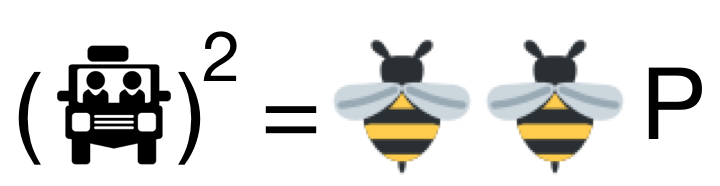# My First Cryptarithm!$\begin{array}{ccccccc} & & & & J & E & E & P\\ \times & & & & J & E & E& P\\ \hline & B & E & E & B & E & E& P \end{array}$

The above shows a cryptarithm such that each letter represents a distinct single non-negative integer with $J, B$ being non-zero. Find the sum of all the possible values of the $4$-digit number $\overline{JEEP}$.

×